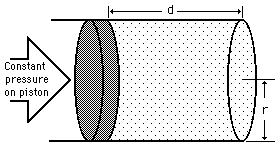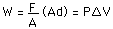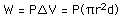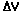# Constant Pressure WorkThe definition of work becomes W=Fd for a constant force F along a distance d. Using the definition of pressure:For the circular piston shown for the case of constant pressure:The calculation of work for this case is straightforward, the main difficulty being the multiplicity of units used for the quantities.

For pressure P = x 10^ Pa = atmos = lb/in^2

if a piston of radius r = m
is moved a distance d = m,
the change in volume is= m^3 = cm^3 = liters,
and the work done on the gas is W = x10^ joules.
 PV diagram for constant pressure process
Index

Heat engine concepts
HyperPhysics***** Thermodynamics Go Back